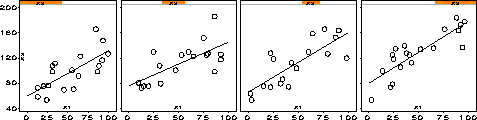coplot Construct a conditioning plot - plots of y * x | z coplot

# SAS Macro Programs: coplot

\$Version: 1.4 (04 Oct 2004)
Michael Friendly
York University

## The coplot macro (get coplot.sas)

### Construct a conditioning plot - plots of y * x | z

The COPLOT macro plots a collection of XY plots, for subsets of a data set conditioned by a GIVEN= variable. These help to show how the relation between X and Y varies with the given variable. A poor-man's version of the Trellis display.

## Usage

The COPLOT macro is defined with keyword parameters. The X=, Y=, and GIVEN= parameters are required. Either or both of the ROWS= and COLS= parameters may be used to arrange the collection of plots in a rectangular grid.

The arguments may be listed within parentheses in any order, separated by commas. For example:

```  %coplot(x=weight, y=mpg, given=Origin);
```

### Parameters

DATA=
Name of the data set to be plotted [Default: `DATA=_LAST_`]
X=
Horizontal variable in each plot
Y=
Vertical variable in each plot
GIVEN=
The name of the slicing variable. May be a numeric or character variable. If numeric, it it treated as continuous, and sliced as determined by the SLICES and OVERLAP parameters. A character GIVEN= variable is treated as discrete, with no OVERLAP between slices.
GROUP=
Grouping variable (determines the plot symbol/color)
SLICES=
Number of slices of the GIVEN= variable [Default: `SLICES=4`]
OVERLAP=
Allowed overlap between slices [Default: `OVERLAP=0.25`]
ROWS=
Number of rows in the panel plot. If neither ROWS= nor COLS= are specified, both values are calculated from the SLICES= value to give a square-ish display. If only one of ROWS= or COLS= is specified, the other value is calculated.
COLS=
Number of columns in the panel plot.
INTERP=
Plot interpolation method used in each panel. E.g., INTERP=RL fits and draws a linear regression line. [Default: `INTERP=NONE`]
HSYM=
Height of plot symbols and value labels in the plots.
GPANEL=
Height of the given panel, in % of the plot size. If `GPANEL=0`, the given panel is suppressed. [Default: `GPANEL=0`]
SYMBOLS=
List of symbols to use for for groups defined by the GROUP= variable. [Default: `SYMBOLS=%STR(CIRCLE SQUARE + : \$ = X _ Y)`]
COLORS=
List of colors to use for for groups. [Default: `COLORS=BLACK RED GREEN BLUE BROWN YELLOW ORANGE PURPLE`]
GOUT=
Name of the output graphics catalog. [Default: `GOUT=GSEG`]

### Limitations

The plots are drawn separately, then composed into one figure with PROC GREPLAY (the PANELS macro). As a result:
• The Given panel does not work very well (font shapes are horribly mangled)
• Axis labels and values for the X and Y variables are not handled very nicely.

### Example

```%include macros(coplot);        *-- or include in an autocall library;

data test;
drop i;
do i=1 to 60;
gp = 1 + mod(i,4);
x1 = round( 100*uniform(0));
x2 = round( 100*uniform(0)) + x1 + 4*gp;
x3 = round( 100*uniform(0)) - x1 + x2 - 4*gp;
output;
end;

%coplot(data=test,
x=X1, y=X2, given=X3, slices=4, overlap=.25,
interp=rl, rows=1);
```panels
scatmat